# Huffman Coding

### Introduction

Huffman coding is a simple method of data compression. In data compression we want to represent some information in the smallest memory space possible. There are two main types of compression:

• Lossy: some data is lost in the process (.jpg, .mp3, etc.)
• Lossless: no data is lost in the process (.png, .flac, .zip etc.)

Huffman coding uses lossless compression.

We will use the following terms when discussing encoding:

• Symbol

A symbol is a representation of a single piece of information.

• Code

A code is the set of possible symbols.

• Sentence

A sentence is a sequence of symbols representing some message.

For example, if we have the code:


{3, 7, 14, 18, 29, 35, 42, 56}

Then the following are sentences:

7, 42, 3, 35, 56, 3
42, 7
3, 3, 3, 35


### Prefix Codes

A prefix code is a set of words with the prefix property. This states that for each word in the code, no valid word is the prefix for another.


{4, 5, 34, 37, 95}

Is a prefix code because none of the words are the prefix of another. However:

{4, 5, 54, 37, 95}


Is not a prefix code because "5" is the prefix of another word "54".

The benefit of a prefix code, is that, given the code, we can identify the individual words without breaks:


Code: {4, 5, 34, 37, 95}
Sentence: 45349553745


What are the symbols in this sentence?

For compressing data, this means we don't have to include separators in the output.

### General Idea

The idea behind Huffman coding is that certain pieces of information in the input are more likely to appear than others. We will give these common symbols shorter codes to conserve space.

For simplicity, we will consider lower-case letters as our words. In English, for example, some letters are much more likely to appear.

This is true for most types of data, allowing for compact representations.

### Binary Tree Representation

A Huffman code works by creating a tree to store the possible symbols. The code used for each symbol is the path from the root to that symbol. For the text:


this is a test

We have the symbols:

{t, h, i, s, a, e}

Then we find the frequency of each:

{t:3, h:1, i:2, s:3, a:1, e:1}


Now we will and create nodes for each symbol: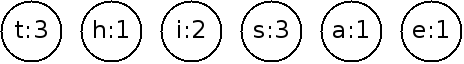### Building The Tree

Next, we will construct the tree by repeatedly joining the nodes with the smallest weights until it is a full tree: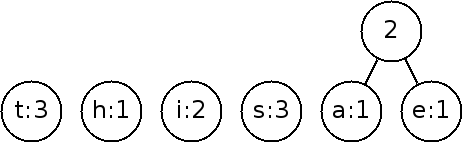The weight of the new node is that of the sum of the children.

By continuing on: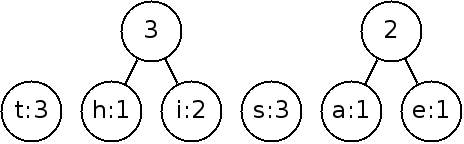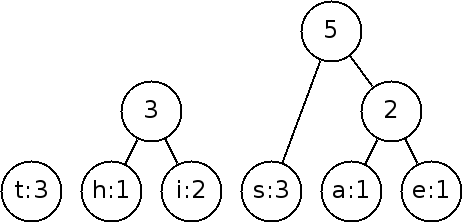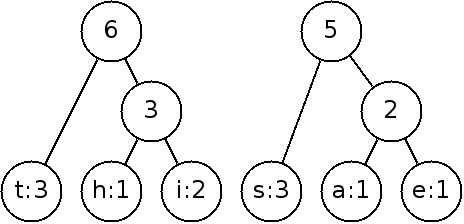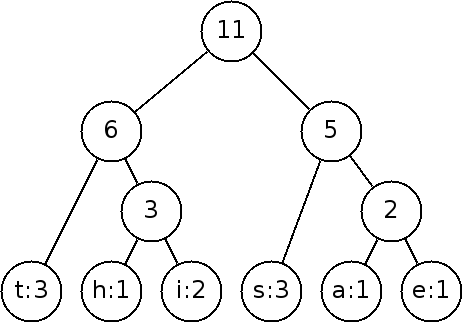To implement this, we need to keep track of the nodes that we can link and choose the smallest weight each time. This is best done with a priority queue (heap)!

• Create a priority queue of nodes such that they are ordered by smallest probability.
• Create a leaf node for each input symbol, and add it to the queue.
• While there is more than one node in the queue:
• Remove the two next nodes from the queue.
• Create a node linking these two as children, with probability of the two children summed.
• Add this node to the queue.
• The one node in the queue is now the root of the complete tree with probability = 1.0

What is the Big-O of this algorithm?

### Finding the Code Words

Now that we have the Huffman tree, the code for each symbol is given by the position in the tree from the root:
• Following the left branch is a "0".
• Following the right branch is a "1".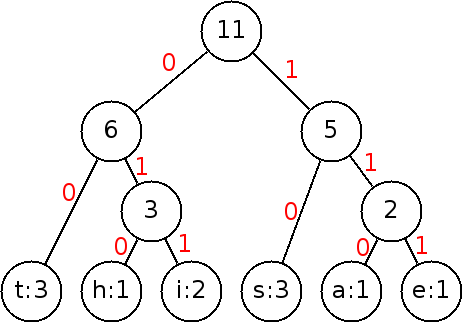This gives us the code:

 Symbol Code t 00 h 010 i 011 s 10 a 110 e 111
Observations:
• This is a prefix code, meaning we don't need to separate words even though it's variable length.
• The two-bit symbols are 't' and 's' the most common, saving space.

In order to find the code for a letter, we need to traverse the tree looking for the letter, keeping track of which path we take.

For efficiency, it's best to find the codes for each letter first, and save them. This can be done recursively:

• Starting at a given node, with a partial code P:
• If this is a letter, save P as the code for this letter and return.
• Recurse to the left with P + "0".
• Recurse to the right with P + "1".

### Compressing Text

To compress text, we simply have to substitute the letter we want with the code it maps to:


t    h     i     s    i     s    a     t    e     s    t
00   010   011   10   011   10   110   00   111   10   00


This seems like it takes more room until you remember characters are usually stored in 8-bit ASCII. With this Huffman code, we can store each letter with 2 or three characters.

Ignoring spaces, we cut the text from 88 bits down to 27 bits.

### Example

This example implements Huffman coding for all lowercase letters. The relative probability of the letters are used to compress English text.

The program uses the algorithms presented above to produce the tree, and find the code for each letter.

It then reads in a file of lower-case letters and writes an output file giving the compressed version.

### Conclusion

A more complete text compression program would also handle:
• Upper-case letters.
• Spaces.
• Symbols & numbers.
• Decompression.

Often, Huffman trees are geared towards a particular type of data where we use all we know about the data to build the tree. This algorithm is often used as the basis for more complex compressions such as jpeg and mp3.